# Python code for MATHEMATICS OF THE DISCRETE FOURIER TRANSFORM (DFT) WITH AUDIO APPLICATIONS

SECOND EDITION

JULIUS O. SMITH III
Center for Computer Research in Music and Acoustics (CCRMA)

#### Python Code by¶

Marina Bosi & Rich Goldberg
Center for Computer Research in Music and Acoustics (CCRMA)

## Spectrum Analysis of a Sinusoid: Windowing, Zero-Padding, and FFT¶

### FFT of a Simple Sinusoid¶

Our first example is an FFT of the simple sinusoid

$\displaystyle x(n) = \cos(\omega_x n T)$

where we choose $\omega_x=2\pi(f_s/4)$ (frequency $f_s/4$ Hz) and $T=1$ (sampling rate $f_s$ set to 1). Since we're using a Cooley-Tukey FFT, the signal length $N$ should be a power of $2$ for fastest results. Here is the Matlab code:

% Example 1: FFT of a DFT-sinusoid

% Parameters:
N = 64;              % Must be a power of two
T = 1;               % Set sampling rate to 1
A = 1;               % Sinusoidal amplitude
phi = 0;             % Sinusoidal phase
f = 0.25;            % Frequency (cycles/sample)
n = [0:N-1];         % Discrete time axis
x = A*cos(2*pi*n*f*T+phi); % Sampled sinusoid
X = fft(x);          % Spectrum

% Plot time data:
figure(1);
subplot(3,1,1);
plot(n,x,'*k');
ni = [0:.1:N-1];     % Interpolated time axis
hold on;
plot(ni,A*cos(2*pi*ni*f*T+phi),'-k'); grid off;
title('Sinusoid at 1/4 the Sampling Rate');
xlabel('Time (samples)');
ylabel('Amplitude');
text(-8,1,'a)');
hold off;

% Plot spectral magnitude:
magX = abs(X);
fn = [0:1/N:1-1/N];  % Normalized frequency axis
subplot(3,1,2);
stem(fn,magX,'ok'); grid on;
xlabel('Normalized Frequency (cycles per sample))');
ylabel('Magnitude (Linear)');
text(-.11,40,'b)');

% Same thing on a dB scale:
spec = 20*log10(magX); % Spectral magnitude in dB
subplot(3,1,3);
plot(fn,spec,'--ok'); grid on;
axis([0 1 -350 50]);
xlabel('Normalized Frequency (cycles per sample))');
ylabel('Magnitude (dB)');
text(-.11,50,'c)');
cmd = ['print -deps ', '../eps/example1.eps'];
disp(cmd); eval(cmd);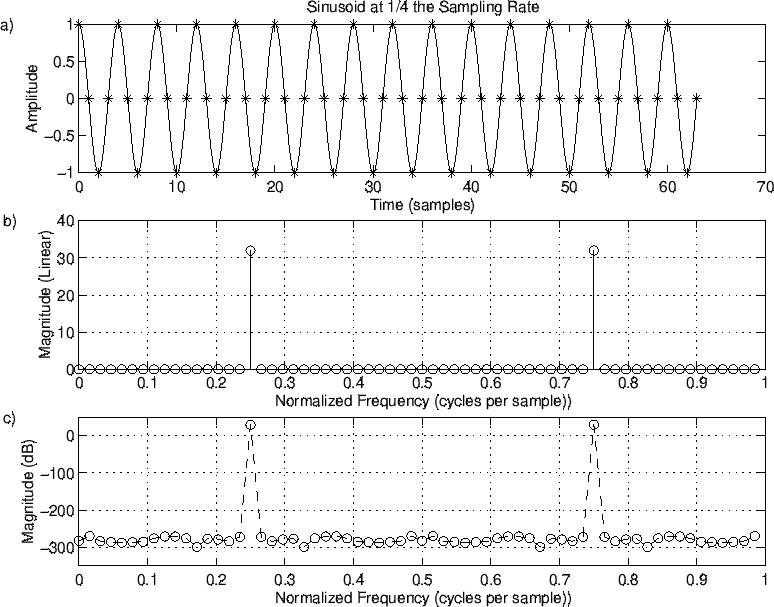Figure 8.1: Sampled sinusoid at frequency $f=f_s/4$ . a) Time waveform. b) Magnitude spectrum. c) DB magnitude spectrum. \includegraphics[width=\twidth]{eps/example1}

The results are shown in Fig.8.1. The time-domain signal is shown in the upper plot (Fig.8.1a), both in pseudo-continuous and sampled form. In the middle plot (Fig.8.1b), we see two peaks in the magnitude spectrum, each at magnitude $32$ on a linear scale, located at normalized frequencies $f= 0.25$ and $f= 0.75 = -0.25$ . A spectral peak amplitude of $32 = (1/2) 64$ is what we expect, since

$$\hbox{DFT}_k(\cos(\omega_x n)) \doteq \sum_{n=0}^{N-1} \frac{e^{j\omega_x n} + e^{-j\omega_x n}}{2} e^{-j\omega_k n},$$

and when $\omega_k=\pm\omega_x$ , this reduces to

$$\sum_{n=0}^{N-1}\frac{e^{j 0 n}}{2} = \frac{N}{2}.$$

For $N=64$ and $\omega_x=2\pi f_s/4$ , this happens at bin numbers $k = 0.25 N = 16$ and $k = 0.75N = 48$ . However, recall that array indexes in matlab start at $1$ , so that these peaks will really show up at indexes $17$ and $49$ in the magX array.

The spectrum should be exactly zero at the other bin numbers. How accurately this happens can be seen by looking on a dB scale, as shown in Fig.8.1c. We see that the spectral magnitude in the other bins is on the order of $300$ dB lower, which is close enough to zero for audio work $(\stackrel{\mbox{.\,.}}{\smile})$ .

In :
# Example 1: FFT of a DFT-sinusoid

import numpy as np
from numpy import pi, cos, log10
from numpy.fft import fft
import matplotlib.pyplot as plt

# Parameters:
N = 64              # Must be a power of two
T = 1               # Set sampling rate to 1
A = 1               # Sinusoidal amplitude
phi = 0             # Sinusoidal phase
f = 0.25            # Frequency (cycles/sample)
n = np.arange(N)    # Discrete time axis
x = A*cos(2*pi*n*f*T+phi) # Sampled sinusoid
X = fft(x)          # Spectrum

plt.figure(figsize=(10,10))

# Plot time data:
plt.subplot(3,1,1)
plt.plot(n,x,'*k')
ni = np.arange(0,N,0.1)   # Interpolated time axis
plt.plot(ni,A*cos(2*pi*ni*f*T+phi),'-k')
plt.xlim(0,N)
plt.title('Sinusoid at 1/4 the Sampling Rate')
plt.xlabel('Time (samples)')
plt.ylabel('Amplitude')
plt.text(-.11*64,1,'a)')

# Plot spectral magnitude:
magX = abs(X)
fn = np.arange(0, 1, 1/N)  # Normalized frequency axis
plt.subplot(3,1,2)
plt.stem(fn,magX,'-ok', use_line_collection=True)
plt.grid()
plt.xlim(0,1)
plt.xlabel('Normalized Frequency (cycles per sample))')
plt.ylabel('Magnitude (Linear)')
plt.text(-.11,30,'b)')

# Same thing on a dB scale:
spec = 20*log10(magX) # Spectral magnitude in dB
plt.subplot(3,1,3)
plt.plot(fn,spec,'--ok')
plt.grid()
plt.xlim(0,1)
plt.ylim(-350, 50)
#plt.axis([0 1 -350 50])
plt.xlabel('Normalized Frequency (cycles per sample))')
plt.ylabel('Magnitude (dB)')
plt.text(-.11,0,'c)')

plt.show()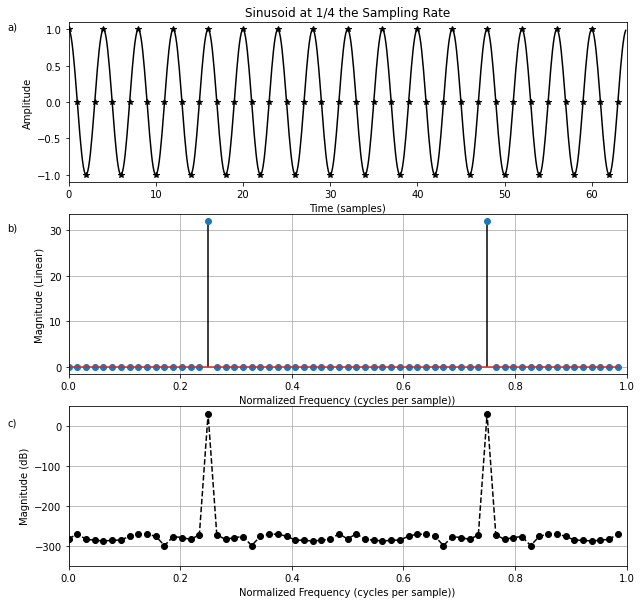### FFT of a Not-So-Simple Sinusoid¶

Now let's increase the frequency in the above example by one-half of a bin:

% Example 2 = Example 1 with frequency between bins

f = 0.25 + 0.5/N;   % Move frequency up 1/2 bin

x = cos(2*pi*n*f*T); % Signal to analyze
X = fft(x);          % Spectrum
...                  % See Example 1 for plots and such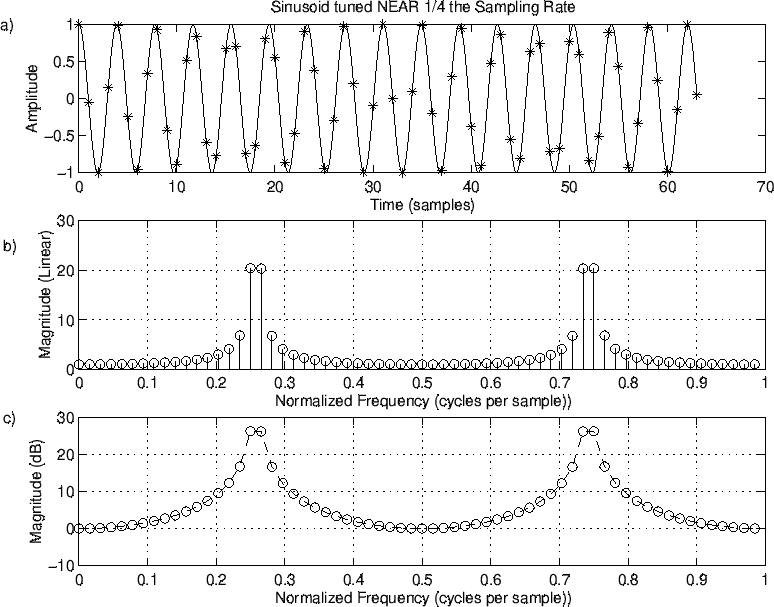Figure 8.2: Sinusoid at Frequency $f=0.25+0.5/N$ . a) Time waveform. b) Magnitude spectrum. c) DB magnitude spectrum.

The resulting magnitude spectrum is shown in Fig.8.2b and c. At this frequency, we get extensive "spectral leakage" into all the bins. To get an idea of where this is coming from, let's look at the periodic extension (§7.1.2) of the time waveform:

% Plot the periodic extension of the time-domain signal
plot([x,x],'--ok');
title('Time Waveform Repeated Once');
xlabel('Time (samples)'); ylabel('Amplitude');

Note the "glitch" in the middle where the signal begins its forced repetition.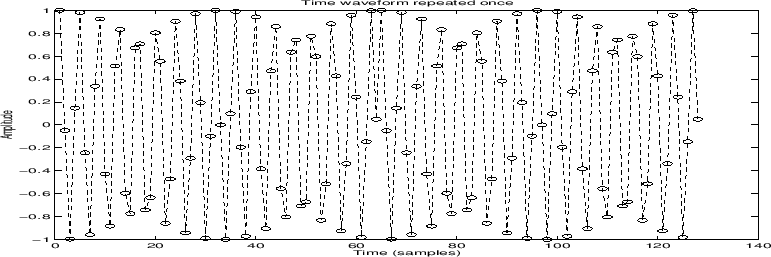Figure 8.3: Time waveform repeated to show discontinuity introduced by periodic extension (see midpoint).

In :
# Example 2 = Example 1 with frequency between bins

import numpy as np
from numpy import pi, cos, log10
from numpy.fft import fft
import matplotlib.pyplot as plt

# Parameters:
N = 64              # Must be a power of two
T = 1               # Set sampling rate to 1
A = 1               # Sinusoidal amplitude
phi = 0             # Sinusoidal phase
#f = 0.25            # Frequency (cycles/sample)
n = np.arange(N)    # Discrete time axis
#x = A*cos(2*pi*n*f*T+phi) # Sampled sinusoid
#X = fft(x)          # Spectrum
f = 0.25 + 0.5/N;    # Move frequency up 1/2 bin
x = cos(2*pi*n*f*T); # Signal to analyze
X = fft(x);          # Spectrum

plt.figure(figsize=(10,10))

# Plot time data:
plt.subplot(3,1,1)
plt.plot(n,x,'*k')
ni = np.arange(0,N,0.1)   # Interpolated time axis
plt.plot(ni,A*cos(2*pi*ni*f*T+phi),'-k')
plt.xlim(0,64)
plt.title('Sinusoid tuned NEAR 1/4 the Sampling Rate')
plt.xlabel('Time (samples)')
plt.ylabel('Amplitude')
plt.text(-.11*64,1,'a)')

# Plot spectral magnitude:
magX = abs(X)
fn = np.arange(0, 1, 1/N)  # Normalized frequency axis
plt.subplot(3,1,2)
plt.stem(fn,magX,'-ok', use_line_collection=True)
plt.grid()
plt.xlim(0,1)
plt.xlabel('Normalized Frequency (cycles per sample))')
plt.ylabel('Magnitude (Linear)')
plt.text(-.11,20,'b)')

# Same thing on a dB scale:
spec = 20*log10(magX) # Spectral magnitude in dB
plt.subplot(3,1,3)
plt.plot(fn,spec,'--ok')
plt.grid()
plt.xlim(0,1)
plt.ylim(-10, 30)
plt.xlabel('Normalized Frequency (cycles per sample))')
plt.ylabel('Magnitude (dB)')
plt.text(-.11,30,'c)')

plt.show()

# Plot the periodic extension of the time-domain signal
plt.figure(figsize=(10,5))
plt.plot(np.concatenate([x,x]),'--ok')
plt.title('Time Waveform Repeated Once')
plt.xlabel('Time (samples)'); plt.ylabel('Amplitude')
plt.show()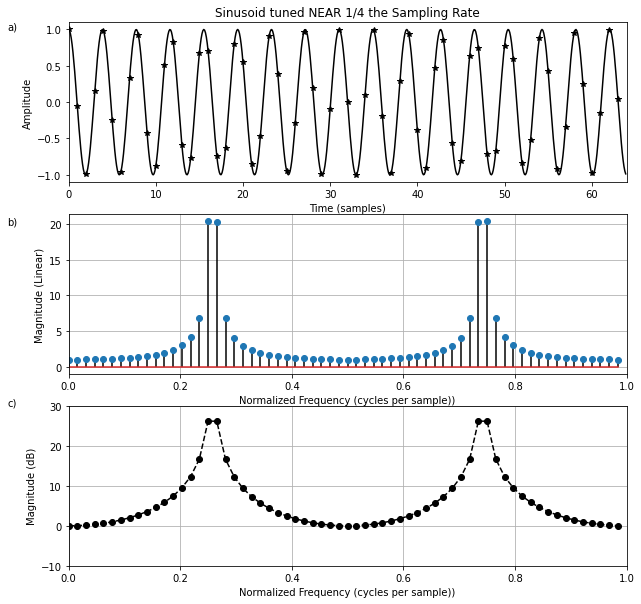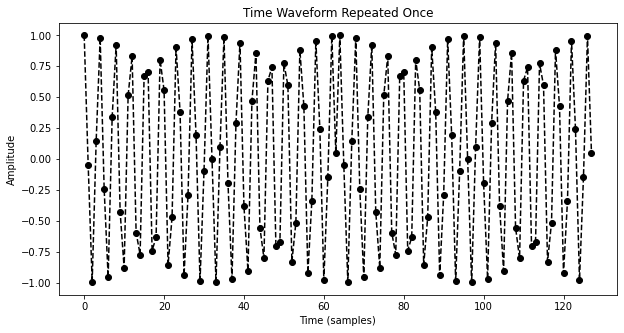### FFT of a Zero-Padded Sinusoid¶

Looking back at Fig.8.2c, we see there are no negative dB values. Could this be right? Could the spectral magnitude at all frequencies be 1 or greater? The answer is no. To better see the true spectrum, let's use zero padding in the time domain (§7.2.7) to give ideal interpolation (§7.4.12) in the frequency domain:

zpf = 8;            % zero-padding factor
X = fft(x);         % interpolated spectrum
magX = abs(X);      % magnitude spectrum
...                 % waveform plot as before
nfft = zpf*N;       % FFT size = new frequency grid size
fni = [0:1.0/nfft:1-1.0/nfft]; % normalized freq axis
subplot(3,1,2);
% with interpolation, we can use solid lines '-':
plot(fni,magX,'-k'); grid on;
...
spec = 20*log10(magX); % spectral magnitude in dB
% clip below at -40 dB:
spec = max(spec,-40*ones(1,length(spec)));
...                 % plot as before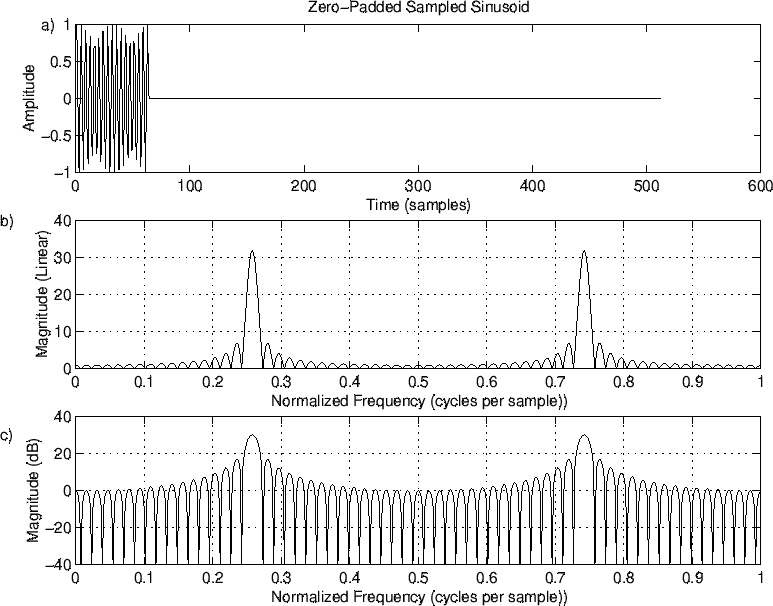Figure 8.4: Zero-padded sinusoid at frequency $f=0.25+0.5/N$ cycles/sample. a) Time waveform. b) Magnitude spectrum. c) DB magnitude spectrum.

Figure 8.4 shows the zero-padded data (top) and corresponding interpolated spectrum on linear and dB scales (middle and bottom, respectively). We now see that the spectrum has a regular sidelobe structure. On the dB scale in Fig.8.4c, negative values are now visible. In fact, it was desirable to clip them at $-40$ dB to prevent deep nulls from dominating the display by pushing the negative vertical axis limit to $-300$ dB or more, as in Fig.8.1c. This example shows the importance of using zero padding to interpolate spectral displays so that the untrained eye will "fill in'" properly between the spectral samples.

In :
zpf = 8            # zero-padding factor
n = np.arange(zpf*N)
x = np.concatenate([cos(2*pi*n[:N]*f*T), np.zeros((zpf-1)*N)]) # zero-padded
X = fft(x)         # interpolated spectrum
magX = abs(X)      # magnitude spectrum

plt.figure(figsize=(10,10))

# Plot time data:
plt.subplot(3,1,1)
plt.plot(n,x,'-k')
plt.xlim(0,N*zpf)
plt.xlabel('Time (samples)')
plt.ylabel('Amplitude')
plt.text(-.11*N*zpf,1,'a)')

# Plot spectral magnitude:
magX = abs(X)
nfft = zpf*N       # FFT size = new frequency grid size
fni = np.arange(0, 1, 1.0/nfft) # normalized freq axis
plt.subplot(3,1,2)
plt.plot(fni,magX,'-k')
plt.grid()
plt.xlim(0,1)
plt.ylim(0, 40)
plt.xlabel('Normalized Frequency (cycles per sample))')
plt.ylabel('Magnitude (Linear)')
plt.text(-.11,40,'b)')

# Same thing on a dB scale:
spec = 20*log10(magX) # Spectral magnitude in dB
plt.subplot(3,1,3)
plt.plot(fni,spec,'-k')
plt.grid()
plt.xlim(0,1)
plt.ylim(-40, 40)
plt.xlabel('Normalized Frequency (cycles per sample))')
plt.ylabel('Magnitude (dB)')
plt.text(-.11,40,'c)')

plt.show()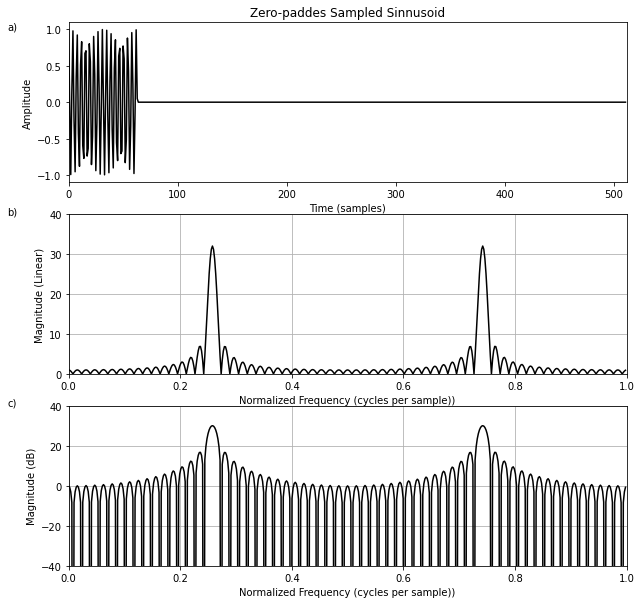### Use of a Blackman Window¶

As Fig.8.4a suggests, the previous example can be interpreted as using a rectangular window to select a finite segment (of length $N$ ) from a sampled sinusoid that continues for all time. In practical spectrum analysis, such excerpts are normally analyzed using a window that is tapered more gracefully to zero on the left and right. In this section, we will look at using a Blackman window on our example sinusoid. The Blackman window has good (though suboptimal) characteristics for audio work.

In Octave or the Matlab Signal Processing Toolbox, a Blackman window of length $M=64$ can be designed very easily:

M = 64;
w = blackman(M);

Many other standard windows are defined as well, including hamming, hanning, and bartlett windows.

In Matlab without the Signal Processing Toolbox, the Blackman window is readily computed from its mathematical definition:

w = .42 - .5*cos(2*pi*(0:M-1)/(M-1)) ...
+ .08*cos(4*pi*(0:M-1)/(M-1));

Figure 8.5 shows the Blackman window and its magnitude spectrum on a dB scale. Fig.8.5c uses the more physical'' frequency axis in which the upper half of the FFT bin numbers are interpreted as negative frequencies. Here is the complete Matlab script for Fig.8.5:

M = 64;
w = blackman(M);
figure(1);
subplot(3,1,1); plot(w,'*'); title('Blackman Window');
xlabel('Time (samples)'); ylabel('Amplitude'); text(-8,1,'a)');

% Also show the window transform:
zpf = 8;                      % zero-padding factor
xw = [w',zeros(1,(zpf-1)*M)]; % zero-padded window
Xw = fft(xw);                 % Blackman window transform
spec = 20*log10(abs(Xw));     % Spectral magnitude in dB
spec = spec - max(spec);      % Normalize to 0 db max
nfft = zpf*M;
spec = max(spec,-100*ones(1,nfft)); % clip to -100 dB
fni = [0:1.0/nfft:1-1.0/nfft];   % Normalized frequency axis
subplot(3,1,2); plot(fni,spec,'-'); axis([0,1,-100,10]);
xlabel('Normalized Frequency (cycles per sample))');
ylabel('Magnitude (dB)'); grid; text(-.12,20,'b)');

% Replot interpreting upper bin numbers as frequencies<0:
nh = nfft/2;
specnf = [spec(nh+1:nfft),spec(1:nh)];  % see fftshift()
fninf = fni - 0.5;
subplot(3,1,3);
plot(fninf,specnf,'-'); axis([-0.5,0.5,-100,10]); grid;
xlabel('Normalized Frequency (cycles per sample))');
ylabel('Magnitude (dB)');
text(-.62,20,'c)');
cmd = ['print -deps ', '../eps/blackman.eps'];
disp(cmd); eval(cmd);
disp 'pausing for RETURN (check the plot). . .'; pause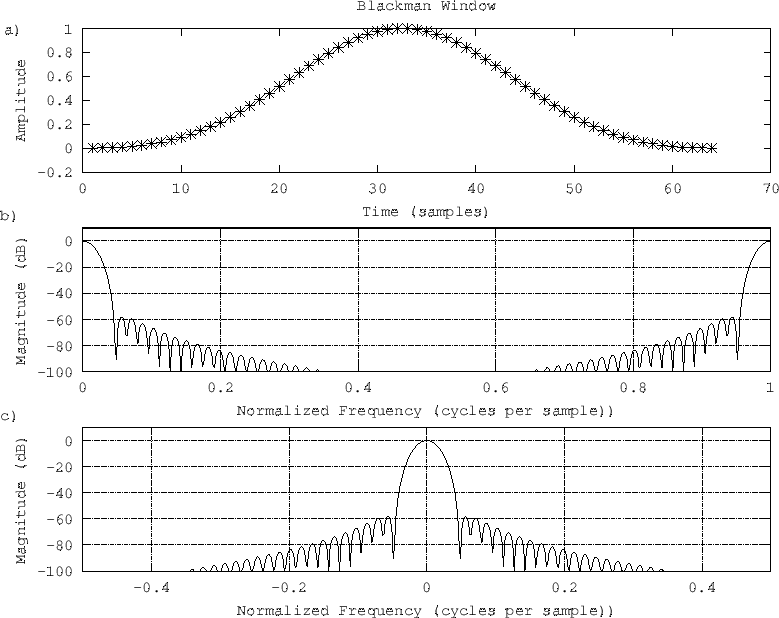Figure 8.5: The Blackman window: a) window itself in the time domain, b) dB magnitude spectrum plotted over normalized frequencies $[0,1)$ , and c) same thing plotted over $[-0.5,0.5)$ .

In :
M = 64
w = np.blackman(M)

plt.figure(figsize=(10,10))

plt.subplot(3,1,1)
plt.plot(w,'-k')
plt.plot(w,'o')
plt.title('Blackman Window')
plt.xlabel('Time (samples)')
plt.ylabel('Amplitude')
plt.text(-8,1,'a)')

# Also show the window transform:
zpf = 8                      # zero-padding factor
xw = np.concatenate([w,np.zeros((zpf-1)*M)] )# zero-padded window
Xw = fft(xw)                 # Blackman window transform
spec = 20*log10(abs(Xw))     # Spectral magnitude in dB
spec = spec - np.max(spec)      # Normalize to 0 db max
nfft = zpf*M
spec = np.maximum(spec,-100*np.ones(nfft)) # clip to -100 dB
fni = np.arange(0, 1, 1/nfft)   # Normalized frequency axis
plt.subplot(3,1,2)
plt.plot(fni,spec,'-')
plt.axis([0,1,-100,10])
plt.xlabel('Normalized Frequency (cycles per sample))')
plt.ylabel('Magnitude (dB)')
plt.grid()
plt.text(-.12,20,'b)')

# Replot interpreting upper bin numbers as frequencies<0:
nh = nfft//2
specnf = np.concatenate([spec[nh:nfft],spec[:nh]])  # see fftshift()
fninf = fni - 0.5
plt.subplot(3,1,3)
plt.plot(fninf,specnf,'-')
plt.axis([-0.5,0.5,-100,10])
plt.grid()
plt.xlabel('Normalized Frequency (cycles per sample))')
plt.ylabel('Magnitude (dB)')
plt.text(-.62,20,'c)')

plt.show()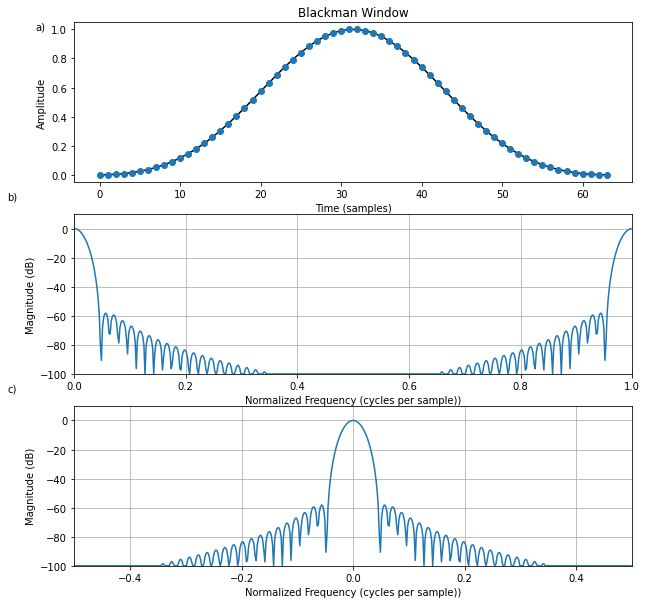#### Applying the Blackman Window¶

Now let's apply the Blackman window to the sampled sinusoid and look at the effect on the spectrum analysis:

% Windowed, zero-padded data:
n = [0:M-1];          % discrete time axis
f = 0.25 + 0.5/M;     % frequency
xw = [w .* cos(2*pi*n*f),zeros(1,(zpf-1)*M)];

% Smoothed, interpolated spectrum:
X = fft(xw);

% Plot time data:
subplot(2,1,1);
plot(xw);
xlabel('Time (samples)');
ylabel('Amplitude');
text(-50,1,'a)');

% Plot spectral magnitude:
spec = 10*log10(conj(X).*X);  % Spectral magnitude in dB
spec = max(spec,-60*ones(1,nfft)); % clip to -60 dB
subplot(2,1,2);
plot(fninf,fftshift(spec),'-');
axis([-0.5,0.5,-60,40]);
title('Smoothed, Interpolated, Spectral Magnitude (dB)');
xlabel('Normalized Frequency (cycles per sample))');
ylabel('Magnitude (dB)'); grid;
text(-.6,40,'b)');

Figure 8.6 plots the zero-padded, Blackman-windowed sinusoid, along with its magnitude spectrum on a dB scale. Note that the first sidelobe (near $-40$ dB) is nearly 60 dB below the spectral peak (near $+20$ dB). This is why the Blackman window is considered adequate for many audio applications. From the dual of the convolution theorem discussed in §7.4.6, we know that windowing in the time domain corresponds to smoothing in the frequency domain. Specifically, the complex spectrum with magnitude displayed in Fig.8.4b has been convolved with the Blackman window transform (dB magnitude shown in Fig.8.5c). Thus, the Blackman window Fourier transform has been applied as a smoothing kernel to the Fourier transform of the rectangularly windowed sinusoid to produce the smoothed result in Fig.8.6b. This topic is pursued in detail at the outset of Book IV in the music signal processing series.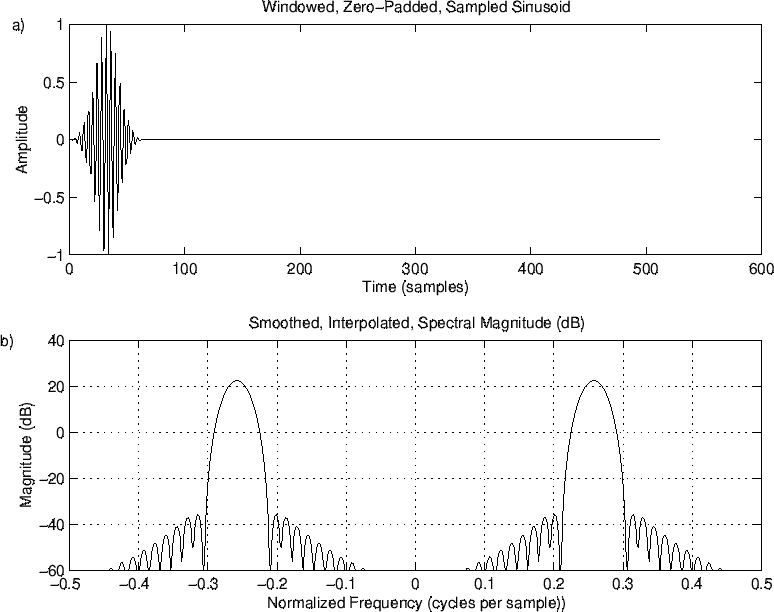Figure 8.6: Effect of the Blackman window on the sinusoidal data.

In :
# Windowed, zero-padded data:
n = np.arange(M)          # discrete time axis
f = 0.25 + 0.5/M     # frequency
xw = np.concatenate([w * cos(2*pi*n*f), np.zeros((zpf-1)*M)] )

# Smoothed, interpolated spectrum:
X = fft(xw)

plt.figure(figsize=(10,10))

# Plot time data:
plt.subplot(2,1,1)
plt.plot(xw)
plt.xlabel('Time (samples)')
plt.ylabel('Amplitude')
plt.text(-80,1,'a)')

# Plot spectral magnitude:
spec = 10*np.log10(X.conjugate() * X).real  # Spectral magnitude in dB
spec = np.maximum(spec,-60 * np.ones(nfft)) # clip to -60 dB
plt.subplot(2,1,2)
plt.plot(fninf,np.fft.fftshift(spec),'-')
plt.axis([-0.5,0.5,-60,40])
plt.title('Smoothed, Interpolated, Spectral Magnitude (dB)')
plt.xlabel('Normalized Frequency (cycles per sample))')
plt.ylabel('Magnitude (dB)')
plt.grid()
plt.text(-.6,40,'b)')

plt.show()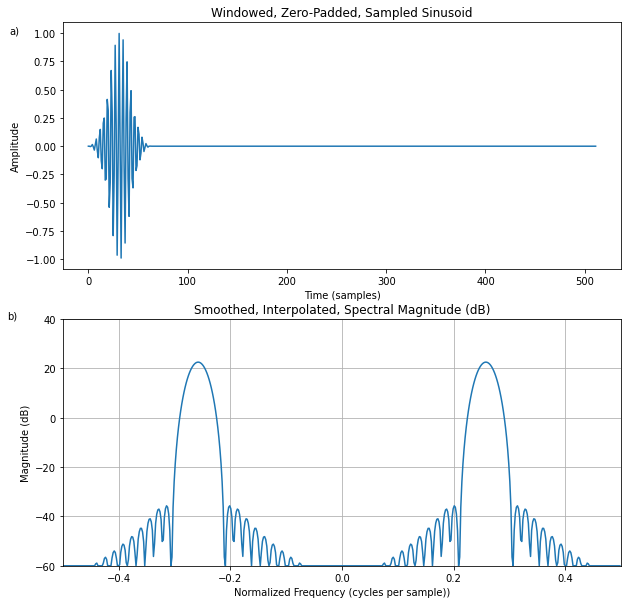### Hann-WindowedComplex Sinusoid¶

In this example, we'll perform spectrum analysis on a complex sinusoid having only a single positive frequency. We'll use the Hann window (also known as the Hanning window) which does not have as much sidelobe suppression as the Blackman window, but its main lobe is narrower. Its sidelobes "roll off" very quickly versus frequency. Compare with the Blackman window results to see if you can see these differences.

The Matlab script for synthesizing and plotting the Hann-windowed sinusoid is given below:

% Analysis parameters:
M = 31;         % Window length
N = 64;         % FFT length (zero padding factor near 2)

% Signal parameters:
wxT = 2*pi/4;   % Sinusoid frequency (rad/sample)
A = 1;          % Sinusoid amplitude
phix = 0;       % Sinusoid phase

% Compute the signal x:
n = [0:N-1];    % time indices for sinusoid and FFT
x = A * exp(j*wxT*n+phix); % complex sine [1,j,-1,-j...]

% Compute Hann window:
nm = [0:M-1];   % time indices for window computation
% Hann window = "raised cosine", normalization (1/M)
% chosen to give spectral peak magnitude at 1/2:
w = (1/M) * (cos((pi/M)*(nm-(M-1)/2))).^2;

wzp = [w,zeros(1,N-M)]; % zero-pad out to the length of x
xw = x .* wzp;          % apply the window w to signal x

figure(1);
subplot(1,1,1);

% Display real part of windowed signal and Hann window
plot(n,wzp,'-k'); hold on; plot(n,real(xw),'*k'); hold off;
title(['Hann Window and Windowed, Zero-Padded, ',...
'Sinusoid (Real Part)']);
xlabel('Time (samples)'); ylabel('Amplitude');

The resulting plot of the Hann window and its use on sinusoidal data are shown in Fig.8.7.